Next: Equilibria of Cylindrical Cloud Up: Hydrostatic Balance Previous: Problem 2   Contents

## Bonnor-Ebert Mass

In the preceding section [Fig.4.1 (left)], we have seen the radial density distribution of a hydrostatic configuration of an isothermal gas. Consider a circumstance that such kind of cloud is immersed in a low-density medium with a pressure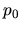. To establish a pressure equilibrium, the pressure at the surface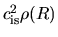must equal to. This means that the density at the surface is constant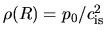.

Figure 4.2 (left) shows three models of density distribution,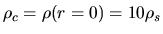,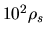, and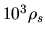. Comparing these three models, it should be noticed that the cloud size (radius) decreases with increasing the central density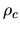. The mass of the cloud is obtained by integrating the distribution, which is illustrated against the central-to-surface density ratio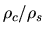in Figure 4.2 (right). The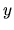-axis represents a normalized mass as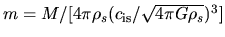. The maximum value of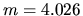means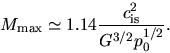(4.9)

This is the maximum mass which is supported against the self-gravity by the thermal pressure with an isothermal sound speed of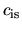, when the cloud is immersed in the pressure. This is called Bonnor-Ebert mass [Bonnor (1956), Ebert (1955)]. It is to be noticed that the critical state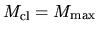is achieved when the density contrast is rather low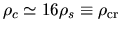.

Another important result from Figure 4.2 (right) is the stability of an isothermal cloud. Even for a cloud with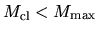, any clouds on the part of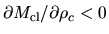are unstable, whose clouds are distributed on the branch with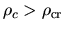. This is understood as follows: For a hydrostatic cloud the mass should be expressed with the external pressure and the central density [Fig.4.2 (right)] as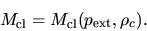(4.10)

In this case, a relation between the partial derivatives such as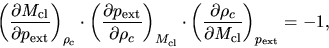(4.11)

is satisfied, unless each term is equal to zero. Figure 4.1 (left) shows that the cloud mass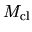is a decreasing function of the external pressure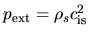, if the central density is fixed. Since this means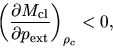(4.12)

equation (4.11) gives us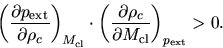(4.13)

For a cloud with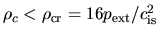the mass is an increasing function of the central density as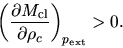(4.14)

Thus, equation (4.13) leads to the relation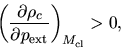(4.15)

for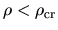. This means that gas cloud contracts (the central density and pressure increase), when the external pressure increases. This is an ordinary reaction of a stable gas.

On the other hand, the cloud on the part of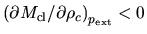(for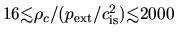) behaves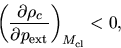(4.16)

and this represents that an extra external pressure decreases the central density and thus the pressure. Pressure encourages expansion. This reaction is unstable.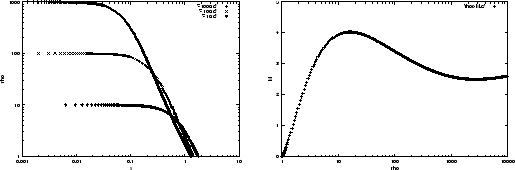Next: Equilibria of Cylindrical Cloud Up: Hydrostatic Balance Previous: Problem 2   Contents
Kohji Tomisaka 2007-07-08